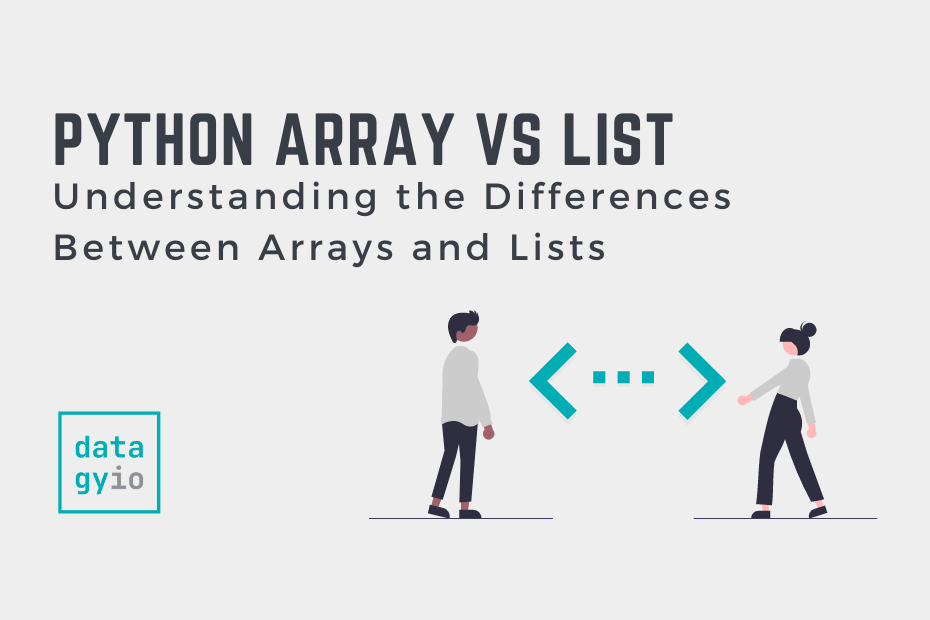# Difference Between Array and List in PythonIn this post, you’ll learn the difference between arrays and lists in Python. Both these data structures let you store data in Python and share many similar properties. However, they also let you do quite different things and knowing when to use which can make you a much stronger programmer!

In particular, you’ll learn how the Python list is different from both the array and the NumPy array. You’ll get a strong understanding of when to use either of these data structures.

By the end of this tutorial, you’ll have learned:

• How Python lists and arrays are similar and how they’re different
• When to use a Python list over an array
• When to use an array over a list in Python

## What is a Python List

A Python list is a built-in data structure that can hold a collection of items. They have a number of unique properties, making them different from other data structures. In particular, Python lists are:

• Ordered – meaning that the items they hold appear in a specific order, allowing you to access the items by their positions
• Mutable – meaning that they items can be added and removed
• Duplicated – meaning that items can exist more than once in the list
• Heterogeneous – meaning that elements can be of different data types, such as strings, integers, and even other lists

Python lists are created using square brackets, `[]`. Let’s create a list containing some numbers:

``````# Creating a Python list is easy!
numbers = [1,2,3,4,5]``````

In the next section, you’ll learn about arrays in Python.

## What is a Python Array

Python comes with a module built-in, `array`, which can be used to create arrays in Python. While arrays maintain most of the characteristics of Python lists, they cannot store items of different data types. They can, however, contain duplicates, are ordered and are mutable.

In order to create an array, we first need to declare it. Generally, this is done by passing in another collection structure, such as a list. When declaring the array, we first need to pass in a data type and then the items we want to store.

Let’s recreate out earlier array:

``````# Creating an array in Python
import array

numbers = array.array('i', [1,2,3,4,5])
print(numbers)

# Returns:
# array('i', [1, 2, 3, 4, 5])``````

## What is a NumPy Array

An array type that you’ll likely encounter more frequently compared to the `array` library’s array, is the NumPy array. The NumPy array is commonly used for numerical calculations. However, it’s much more akin to the Python list. In fact, it carries all of the same properties of a Python list (including storing different data types). Because NumPy is intended to be used for numerical calculations, it also comes with many different methods and functions to support this.

Let’s see how we can create a NumPy array containing the same items as our original list:

``````# Creating a NumPy Array
import numpy as np

numbers = np.array([1,2,3,4,5])
print(numbers)

# Returns:
# [1 2 3 4 5]``````

Similar to the array from the `array` library, we need to declare the NumPy array. However, because these arrays can contain different data types, we don’t need to declare the data type first.

## Python List vs Array Compared

In this section, we’ll compare the Python list, the array, and the NumPy array:

## When to Use a List Over an Array in Python

You should use a Python list over an array when you are simply wanting to store a small collection of items to which you don’t want to perform any mathematical operations. Because Python lists are easily created and mutated, they make great candidates for these types of operations.

However, if you want to perform mathematical operations, the work becomes a bit trickier. Let’s image we wanted to add 5 to our original list `numbers`. We would need to use either a for loop or a list comprehension to add the value, element by element.

Let’s see what this looks like:

``````# Adding Values to List Items
numbers = [1,2,3,4,5]
new_numbers = []
for number in numbers:
new_numbers.append(number + 5)

print(new_numbers)

# Returns:
# [6, 7, 8, 9, 10]``````

## When to use an Array over a List in Python

You should choose to use an array over a list in Python if you know that you will need to perform mathematical operations to it. Similarly, if you need to store complex data of similar structure (such as matrices), this can be a more memory-efficient process.

Let’s see how easy it is to replicate our early example using a NumPy array:

``````# Adding Values to Array Items
import numpy as np
numbers = np.array([1,2,3,4,5])
numbers += 5

print(numbers)

# Returns:
# [ 6  7  8  9 10]``````

## Conclusion

In this tutorial, you learned the differences between Python lists and the two types of arrays available in Python: the array library and the NumPy library. You then learned how they are similar and what they do differently. Finally, you learned when to use which option, specifically targeting different use cases